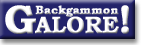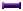Cube Handling in RacesKleinman count

 From: Řystein Johansen Address: ojohans@statoil.com Date: 22 February 2001 Subject: Re: Kleinman Count Forum: rec.games.backgammon Google: 3A9531C7.A6D9BE0B@statoil.com

```Michael Crane wrote:
> I'm looking for en explanation of the 'Kleinman Count'. I understand
> it is for avoiding leaving blots when rolling large doubles ... but
> I could be wrong!

I believe 'Kleinman Count' is the method of estimating your game winning
probability in a race, based on the pip-count.

K is calculated by this equation:

K = D^2 / S                  (Eq. 1)

where D = the difference of pip-count of the two players
S = the sum of each players pip-counts
(Subtract 4 to compensate for the player on roll pips)

If K > 1.0

W = 0.76 + 0.114 * log (K)   (Eq. 2)

(log(K) refers to the natural logarithm)

If K < 1.0

W = 0.5 + 0.267 * sqrt(K)    (Eq. 3)

Where W = game winning probability in race.

Danny Kleinman did not give us equation 2 and 3, but gives a table of
winning probabilities for the different values of K. Equation 2 is my
own little regression analyses, and equation 3 is the work of Chuck
Bower .

Example 1:
I'm on roll got 100 pips in the race, you 113 pips. What's my
probability to win the race?

D = 113 - 100 + 4 = 17
S = 113 + 100 - 4 = 209

K = 17 * 17 / 209 = 1.383

W = 0.76 + 0.114 * log(1.383) = 0.797

So, the Kleinman Count estimates a winning probability in the race of
79.7%

Example 2:
I'm on roll got 100 pips in the race, you 106 pips. What's my
probability to win the race?

D = 106 - 100 + 4 = 10
S = 106 + 100 - 4 = 202

K = 10 * 10 / 202 = 0.495

W = 0.5 + 0.267 * sqrt(0.495) = 0.688

So, the Kleinman Count estimates a winning probability in the race of
68.8%

Now comes the big questions: Can you do this math in your head over the
board?
The answer is no, maybe someone can, but I can't, and I guess most of
the readers can't either. But there is some of this math that can be
done in your head over the board. Calculating the K should be possible
usually find a good value for the division to get K.

Remember some key values of K and assign the winning probability for
this values. That's all you need to remember! Forget about the square
roots and logarithms.

K = 0.5   =>  W = 0.69  (a double)
K = 0.7   =>  W = 0.72  (a redouble)

K = 1.0   =>  W = 0.76  (a take)
K = 1.2   =>  W = 0.78  (borderline take/drop)
K = 1.4   =>  W = 0.80
K = 2.0   =>  W = 0.84

Chuck Bower has a more complete table in his posting .

I have also implemented these equations as C code:
http://subversions.gnu.org/cgi-bin/cvsweb/~checkout~/gnubg/kleinman.c?rev=1.1&content-type=text/plain

Best regards,
Řystein Johansen  8^)

Chuck Bowers article:
 http://www.bkgm.com/rgb/rgb.cgi?view+500
```

 Řystein Johansen  writes: ```Yes, I wrote that, but I can see further now. Kleinman developed this model with some simple assumption. The average pip of a roll is 8. The standard deviation of a roll is 4.3. Let's say about 4.0. Being on roll is worth 4 pips. Kleinman uses the following figures: T = 'True shakers pips' --- The pip count of player on roll minus 4 N = the pip count of the player not on roll. S = N + T --- The length of the race. D = N - T --- The difference. So, if you have a race of S pips then you will use about S/8 shakes to get off. To get standard deviation, we can add up the variance of each roll. The variance is defined as the the std.deviation squared. If the standard deviation of each roll is assumed 4, so the variance of each roll must be 16. The standard deviation of pips over S/8 rolls must then be: std.dev = sqrt( (S/8) * 16 ) = sqrt( 2S ) Now find a table over the standard cumulative normal distribution. You can find it in most books of statistics, usually in the appendix, Now take the ratio D/sqrt(2S). Look up this number in the table. The number you find there is the probability that the player on roll will win. Amazing! How is this table calculated: x / 2 P( X <= x ) = | ( 1 / sqrt(2 *pi) ) * exp( -X / 2 ) dX / -inf I must admit I don't use this formula over the board. I don't know Danny personally, but I'm quite sure he doesn't either. He even suggest that the ratio D/sqrt(2S) is squared. This should be done to eliminate the square root operation. The expression to calculate over the board will then be (D^2)/2S. Much simpler, and then he sets up a table based on this ratio instead. Now that you know the theory behind this, you can set up your own table of winning probabilities, and post the results here on r.g.b. -Řystein ```

### Cube Handling in Races

Bower's modified Thorp count  (Chuck Bower, July 1997)
Calculating winning chances  (Raccoon, Jan 2007)
Calculating winning chances  (OpenWheel+, Nov 2005)Doubling formulas  (Michael J. Zehr, Jan 1995)
Doubling in a long race  (Brian Sheppard, Feb 1998)
EPC example: stack and straggler  (neilkaz+, Jan 2009)
EPC examples: stack and straggler  (Carlo Melzi+, Dec 2008)
Effective pipcount  (Douglas Zare, Sept 2003)Effective pipcount and type of position  (Douglas Zare, Jan 2004)Kleinman count  (Øystein Johansen+, Feb 2001)
Kleinman count  (André Nicoulin, Sept 1998)
Kleinman count  (Chuck Bower, Mar 1998)Lamford's race forumla  (Michael Schell, Aug 2001)
N-roll vs n-roll bearoff  (David Rubin+, July 2008)
N-roll vs n-roll bearoff  (Gregg Cattanach, Nov 2002)
N-roll vs n-roll bearoff  (Chuck Bower+, Dec 1997)
Near end of game  (Daniel Murphy, Mar 1997)
Near end of game  (David Montgomery, Feb 1997)
Near end of game  (Ron Karr, Feb 1997)
One checker model  (Kit Woolsey+, Feb 1998)
Pip count percentage  (Jeff Mogath+, Feb 2001)
Pip-count formulas  (Tom Keith+, June 2004)Thorp count  (Chuck Bower, Jan 1997)
Thorp count  (Simon Woodhead, Sept 1991)
Thorp count questions  (Chuck Bower, Sept 1999)
Value of a pip  (Tom Keith, June 2004)
Ward's racing formula  (Marty Storer, Jan 1992)
What's your favorite formula?  (Timothy Chow+, Aug 2012)From GammOnLineLong messageRecommended readingRecent addition

 Book Suggestions Books Cheating Chouettes Computer Dice Cube Handling Cube Handling in Races Equipment Etiquette Extreme Gammon Fun and frustration GNU Backgammon History Jellyfish Learning Luck versus Skill Magazines & E-zines Match Archives Match Equities Match Play Match Play at 2-away/2-away Miscellaneous Opening Rolls Pip Counting Play Sites Probability and Statistics Programming Propositions Puzzles Ratings Rollouts Rules Rulings Snowie Software Source Code Strategy--Backgames Strategy--Bearing Off Strategy--Checker play Terminology Theory Tournaments Uncategorized Variations